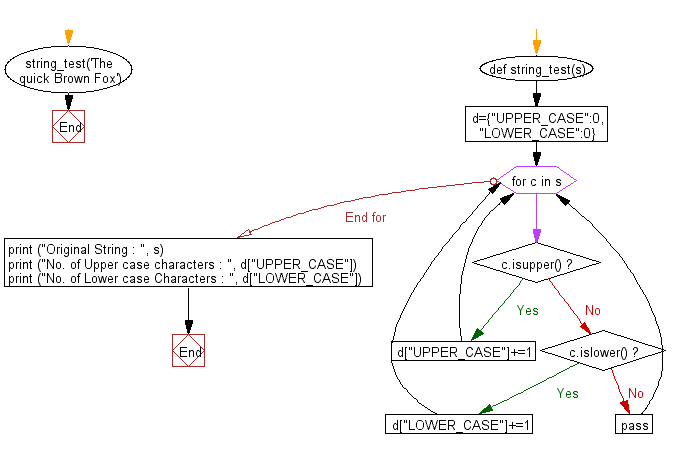﻿ Python Exercise: Calculate the number of upper / lower case letters in a string - w3resource# Python Exercise: Calculate the number of upper / lower case letters in a string

## Python Functions: Exercise-7 with Solution

Write a Python function that accepts a string and calculate the number of upper case letters and lower case letters.

Sample Solution:-

Python Code:

``````def string_test(s):
d={"UPPER_CASE":0, "LOWER_CASE":0}
for c in s:
if c.isupper():
d["UPPER_CASE"]+=1
elif c.islower():
d["LOWER_CASE"]+=1
else:
pass
print ("Original String : ", s)
print ("No. of Upper case characters : ", d["UPPER_CASE"])
print ("No. of Lower case Characters : ", d["LOWER_CASE"])

string_test('The quick Brown Fox')
```
```

Sample Output:

```Original String :  The quick Brow Fox
No. of Upper case characters :  3
No. of Lower case Characters :  13
```

Pictorial presentation:Flowchart:## Visualize Python code execution:

The following tool visualize what the computer is doing step-by-step as it executes the said program:

Python Code Editor:

Have another way to solve this solution? Contribute your code (and comments) through Disqus.

What is the difficulty level of this exercise?

Test your Programming skills with w3resource's quiz.

﻿

## Python: Tips of the Day

Decapitalizes the first letter of a string:

Example:

```def tips_decapitalize(s, upper_rest=False):
return s[:1].lower() + (s[1:].upper() if upper_rest else s[1:])
print(tips_decapitalize('PythonTips'))
print(tips_decapitalize('PythonTips', True))
```

Output:

```pythonTips
pYTHONTIPS
```